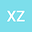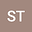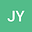Riemann-Hilbert approach and N-soliton solutions for a new four-component nonlinear Schrödinger equation
•••• +1
• Xin-Mei Zhou,
• Shou-Fu Tian,
• Jin-Jie Yang,
• Jin-Jin Mao
Xin-Mei Zhou
China University of Mining and Technology
Author ProfileShou-Fu Tian
China University of Mining and Technology
Author ProfileJin-Jie Yang
China University of Mining and Technology
Author ProfileJin-Jin Mao
China University of Mining and Technology
Author Profile## Abstract

A new four-component nonlinear Schrödinger equation is first proposed in this work and studied by Riemann-Hilbert approach. Firstly, we derive a Lax pair associated with a $5\times5$ matrix spectral problem for the four-component nonlinear Schrödinger equation. Then based on the Lax pair, we analyze the spectral problem and the analytical properties of the Jost functions, from which the Riemann-Hilbert problem of the equation is successfully established. Moreover, we obtain the $N$-soliton solutions of the equation by solving the Riemann-Hilbert problem without reflection. Finally, we derive two special cases of the solutions to the equation for $N=1$ and $N=2$, and the local structure and dynamic behavior of the one-and two-soliton solutions are analyzed graphically.